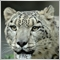# EA - vertically scaling chart in mql481

I need to scale the chart to maximum(or something below a bit below) in mql4.

like when you put your mouse over the bar on the right and drag upwards.

`ChartSetInteger(0,CHART_SCALE,0,5);`

but this only zooms the chart and leaves the scaling as is.882

Hello friend,

I'm not sure, but I think that you mean this

```ChartSetInteger(0,CHART_AUTOSCROLL,true);
ChartSetInteger(0,CHART_SCALE,3);

```

Btw "CHART_SCALE=0" is like the size of the bars on the chart. There as "CHART_AUTOSCROLL,true" is moving the chart across to the left.

`ChartSetInteger(0,CHART_SHIFT,1);`
"CHART_SHIFT" moves the bars to the left.81

GrumpyDuckMan:

Hello friend,

I'm not sure, but I think that you mean this

Btw "CHART_SCALE=0" is like the size of the bars on the chart. There as "CHART_AUTOSCROLL,true" is moving the chart across to the left.

"CHART_SHIFT" moves the bars to the left.

hello friend :)

but as stated I already tried CHART_SCALE property and just tested again with your other suggestions  and it does zoom alright as expected,

BUT(!) I'm looking for a way to set the scaling as if you would be placing your mouse

on the price bar to the right, press left mouse button and drag upwards or use scrollwheel.

this changes scaling beyond the CHART_SCALE approach.

I need a way to set this other scaling - not(!) zooming 0-5 - in mql222

Have a look how this indicator is doing it....

```//+------------------------------------------------------------------+
//|                                             Auto scale chart.mq4 |
//+------------------------------------------------------------------+
#property version   "1.00"
#property strict
#property indicator_chart_window

extern string Explanation= "Chart Space is a percent of the vertical axis as blank space above & below price";
extern string Inputs= "Input percent number between 0 and 49 below";
extern int chart_space= 20;
bool ChartSetInteger();
bool ChartSetDouble();

//+------------------------------------------------------------------+
//| Custom indicator initialization function                         |
//+------------------------------------------------------------------+
int OnInit()
{
//---
Comment("");
if(chart_space<0 || chart_space>49)
{
Comment("Please enter chart_space value between 0 and 49");
Print("Please enter chart_space value between 0 and 49");
return(0);
}
ChartSetInteger(0,CHART_SCALEFIX,TRUE);
//---
return(INIT_SUCCEEDED);
}
//+------------------------------------------------------------------+
//| Custom indicator deinitialization function                       |
//+------------------------------------------------------------------+
int deinit()
{
//----
Comment("");
ChartSetInteger(0,CHART_SCALEFIX,FALSE);
//----
return(0);
}
//+------------------------------------------------------------------+
//| Custom indicator iteration function                              |
//+------------------------------------------------------------------+
int OnCalculate(const int rates_total,
const int prev_calculated,
const datetime &time[],
const double &open[],
const double &high[],
const double &low[],
const double &close[],
const long &tick_volume[],
const long &volume[],

{
//---
int iLeft   = WindowFirstVisibleBar();
int iRight  = iLeft-WindowBarsPerChart();
if(iRight < 0) iRight = 0;     // Chart shifted

double bars_price_max = High[iHighest(NULL,0,MODE_HIGH,iLeft-iRight+1,iRight)];
double bars_price_min = Low[ iLowest(NULL,0,MODE_LOW, iLeft-iRight+1,iRight)];

double chart_price_max= bars_price_max + (( bars_price_max - bars_price_min ) /(100-2*chart_space)*chart_space);
double chart_price_min= bars_price_min - (( bars_price_max - bars_price_min ) /(100-2*chart_space)*chart_space);

ChartSetDouble(0,CHART_FIXED_MAX,chart_price_max);
ChartSetDouble(0,CHART_FIXED_MIN,chart_price_min);

//--- return value of prev_calculated for next call
return(rates_total);
}
//+------------------------------------------------------------------+```